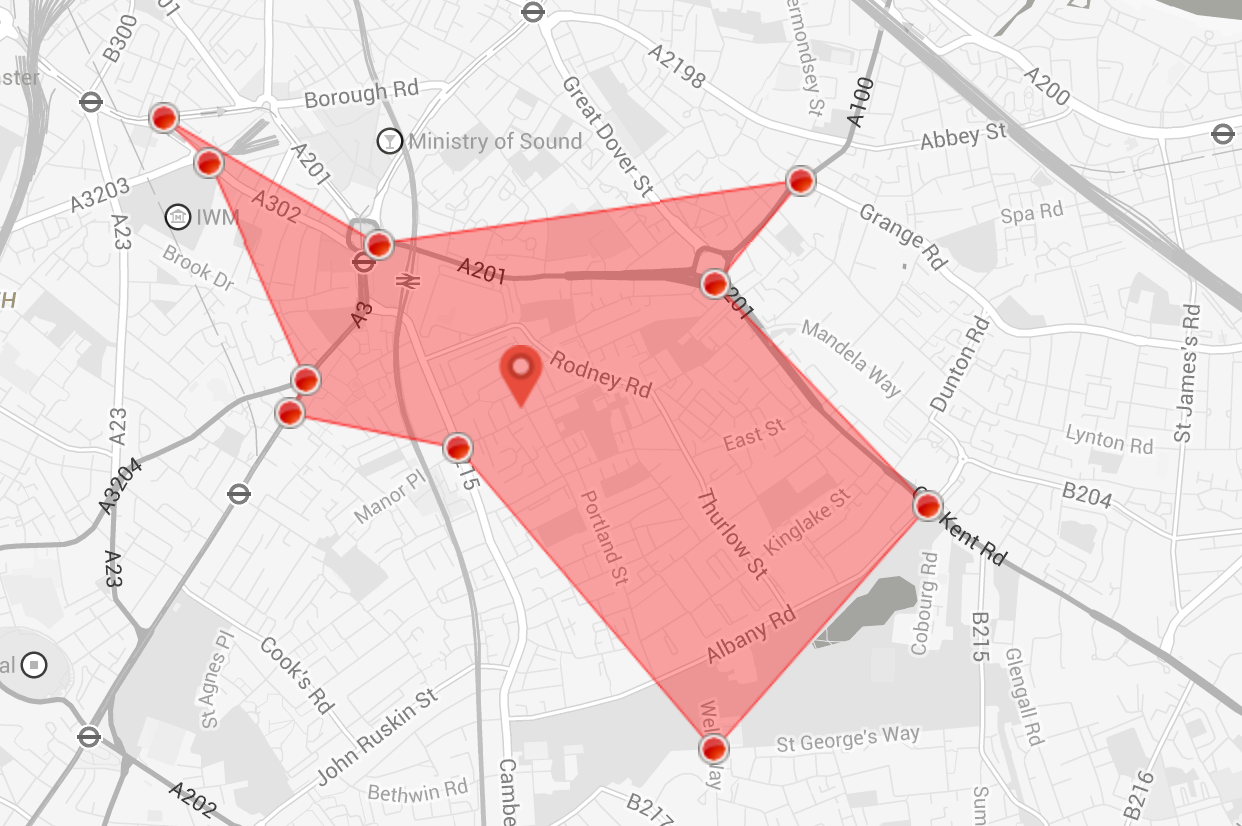IntroductionIsochrones are curves of equal travel time from a certain point of origin, another way of saying this would be that an Isochrone is an isoline for travel time, if the weighting factor is changed form time to distance, then the resulting curve us called an Isodistance.

In this post I will present a rough way to create an Isochrone and an Isodistance using google’s directions API.

Algorithm

The actual algorithm to calculate Isocrone or Isodistance is perhpas a bit complicated than the simplistic approach being described here, if you are intrested in having a deeper look then I would recommend visiting this link by OpenStreetMap folks. Here is a link to my version on github. Open Isochrone.html to take a look at the functionality or visit this hosted link.

Details

Since we do not have direct access to road network (to crawl minimum spanning tree), we fist start the process by drawing a circle of x meters for Isodistance or make a fair assumption for Isochrones. Following code describes the process. ``` function getCirclePoints(center, radius) {

var circlePoints = [];
var searchPoints = [];
with(Math) {
var rLat = (radius / 6378.135) * (180 / PI);
var rLng = rLat / cos(center.lat() * (PI / 180));
for (var a = 0; a < 361; a++) {
var aRad = a * (PI / 180);
var x = center.lng() + (rLng * cos(aRad));
var y = center.lat() + (rLat * sin(aRad));
var point = new google.maps.LatLng(parseFloat(y), parseFloat(x));
circlePoints.push(point);
if (a % pointInterval == 0) {
searchPoints.push(point)
}
}
}
paths: circlePoints,
strokeColor: '#000000',
strokeOpacity: 1,
strokeWeight: 1,
fillColor: '#ffffff',
geodesic: true,
fillOpacity: 0.5,
clickable: false
});
searchPolygon.setMap(map);
map.fitBounds(searchPolygon.getBounds());
return searchPoints

}

getCirclePoints method uses a point interval of 5 and returns around 72 points (360/5), all around the circumference of the circle. Next, we use the center of the circle as our starting point, and call direction services for each of the point in the circumference. ```
var from = startpoint.lat() + ' ' + startpoint.lng();
var to = searchPoints.lat() + ' ' + searchPoints.lng();

//Removed processed Point.
searchPoints.shift()

var request = {
origin: from,
destination: to,
avoidHighways: false
};

directionsService.route(request, directionsearch)

Steps

For each of the requested responses, code traverse through the steps in the legs, then depending on the weighing factor we compute the desired distance or time as shown below ``` for (var n = 0; n < steps.length; n++) {

if(ISOCHRONE)
unit += steps[n].duration.value;
else
unit += steps[n].distance.value;

if (unit < comparator) {
temp\_Points.push(steps[n].end\_location)
}
else {
break;
}
}
The whole process can be observed by directions debug lines. ![Isochrone](http://varunpant.com/static/resources/isochrone.gif)